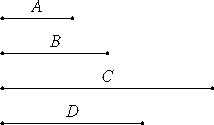# Proposition 5

If a cubic number multiplied by any number makes a cubic number, then the multiplied number is also cubic.

Let the cubic number A multiplied by any number B make the cubic number C.

I say that B is cubic.IX.3

Multiply A by itself to make D. Then D is a cube.

VII.17

Now, since A multiplied by itself makes D, and multiplied by B makes C, therefore A is to B as D is to C.

And since D and C are cubes, therefore they are similar solid numbers. Therefore two mean proportional numbers fall between D and C. And D is to C as A is to B, therefore two mean proportional numbers fall between A and B, too.

VIII.23

And A is a cube, therefore B is also a cube.

Therefore, if a cubic number multiplied by any number makes a cubic number, then the multiplied number is also cubic.

Q.E.D.

## Guide

This proposition is a converse of the previous one. When ab = c, and a is a cube, the previous proposition said that if b is a cube, then c is also, while this proposition says that if c is a cube, then b is also.